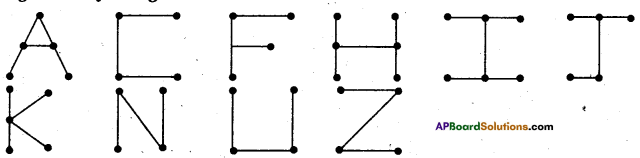AP State Syllabus AP Board 6th Class Maths Solutions Chapter 8 Basic Geometric Concepts Ex 8.1 Textbook Questions and Answers.

## AP State Syllabus 6th Class Maths Solutions 8th Lesson Basic Geometric Concepts Ex 8.1Question 1.
In the given figure there are some points marked. Name them.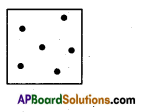Solution: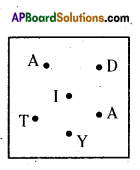Question 2.
Join the points given below. Name the line segments so formed in the figure.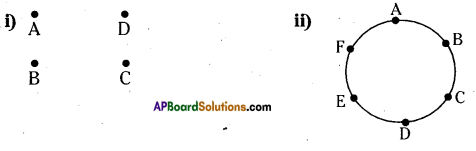Solution: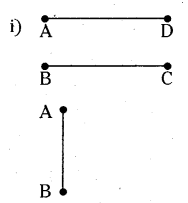$$\overline{\mathrm{AD}}$$ read as segment AD
$$\overline{\mathrm{BC}}$$ read as segment BC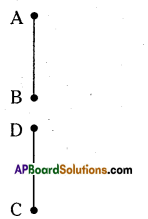$$\overline{\mathrm{AB}}$$ read as segment AB

$$\overline{\mathrm{DC}}$$ read as segment DC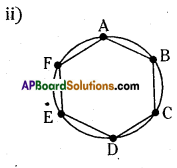$$\overline{\mathrm{AB}}$$ read as segment AB
$$\overline{\mathrm{BC}}$$ read as segment BC
$$\overline{\mathrm{CD}}$$ read as segment CD
$$\overline{\mathrm{DE}}$$ read as segment DE
$$\overline{\mathrm{EF}}$$ read as segment EF
$$\overline{\mathrm{FA}}$$ read as segment FAQuestion 3.
Identify the following from the given figure.
(i) Any six points.
(ii) Any six line segments. (Starts with G)
(iii) Any six rays. (With initial point I)
(iv) Any three lines.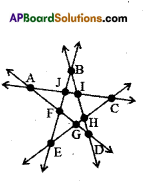Solution:
Points are : A, B, C, D, E, F
G, H, I, and J
ii) Line segments are : $$\overline{\mathrm{GF}}, \overline{\mathrm{GE}}, \overline{\mathrm{GD}}, \overline{\mathrm{GH}}, \overline{\mathrm{GC}} \text { and } \overline{\mathrm{GA}}$$
iii) Rays are: $$\overrightarrow{\mathrm{IA}}, \overrightarrow{\mathrm{IC}}, \overrightarrow{\mathrm{IB}}, \overrightarrow{\mathrm{ID}}, \overrightarrow{\mathrm{JA}}, \overrightarrow{\mathrm{JC}}, \overrightarrow{\mathrm{JE}}$$
iv)Lines are :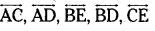Question 4.
Write ‘True’ or ‘False’.
(i) A line has two end points.
(ii) Ray is a part of line.
(iii) A line segment has two end points.
(iv) We can draw many lines through two points.
Solution:
i) A line has two end points. (False)
ii) Ray is a part of line.(True)
iii) A line segment has two end points.(True)
iv) We can draw many lines through two points.(False)Question 5.
Draw and Name :
(T) Line containing point K.
QS) Draw a circle and a line such that the line intersects the circle.
a) at no points b) at one point c) at two points
(iii) Can we draw a line and a circle with 3 intersecting points ?
Solution: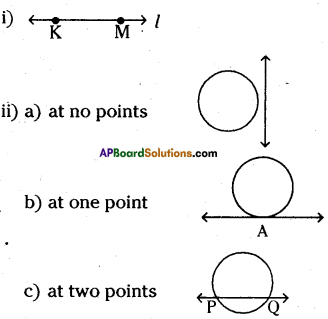iii) No, we can’t draw a line and a circle with 3 intersecting points. As a line can intersect the circle at two points only.

Question 6.
List out all capital letters in English alphabet, that you can write with 3 line segments by using all.
Solution: